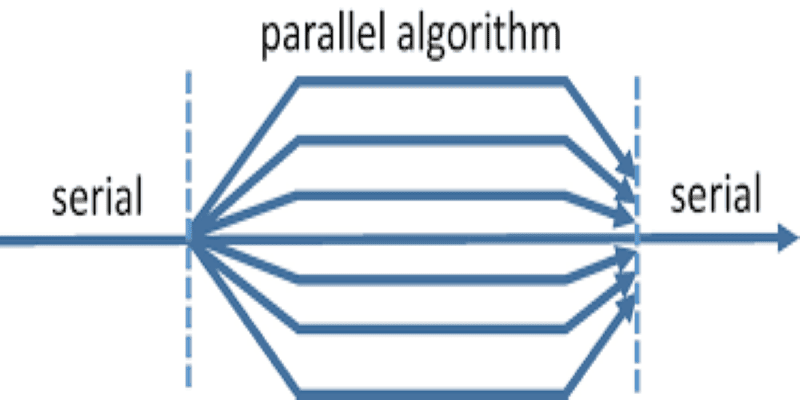0(0)

# Parallel Algorithms

• Course level: Beginner

#### Parallel Algorithms. A conventional algorithm uses a single processing element. A parallel algorithm assumes that there are multiple processors.

These processors may communicate with each other using shared memory or an interconnection network. An algorithm designed for a large number (for example, a polynomial in the problem size) of processors can be simulated on a machine with a small number of a processor for a trade-off on time, and therefore is of practical value, while at the same time allowing us to test the limits of parallelism.

Many algorithmic design techniques in the parallel setting will be explored. Parallel complexity theory will also be briefly studied.

#### What Will I Learn?

• Week 1: Theoretical models: PRAM, interconnection networks
• Week 2: Performance of parallel algorithms, Basic techniques
• Week 3: Basic techniques
• Week 4: Comparator Networks.
• Week 5: Optimal List ranking, applications
• Week 6: Algorithms for searching, merging, and sorting. Cole’s Merge Sort
• Week 7: Cole’s Merge Sort(cont’d), Graph algorithms
• Week 8: Graph algorithms (cont’d)
• Week 9: Sorting in meshes, Hypercube algorithms, Butterfly network, CCC, Benes network
• Week 10: Butterfly network, CCC, Benes network etc
• Week 11: Limits to parallelizability. Lower bounds
• Week 12: Limits to parallelizability. NC-reductions, P-completeness.

38 Lessons

#### Parallel Algorithms

##### Lec 37: Max Flow is P-complete for NC-reductions00:00:00Free